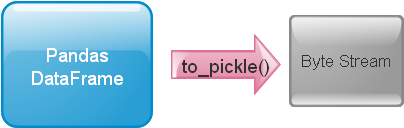# to_pickle()

 `path ( file ) ` Path with file name to store the byte string of pickled data `compression` (Optional )Type of compression, {'infer', 'gzip', 'bz2', 'zip', 'xz', None} `protocol` (Optional) , Int, we can use HIGHEST_PROTOCOL to get the latestWe can create a file with DataFrame data by using to_pickle(). We will create one DataFrame by using a dictionary.
What is pickle ?
Python Pandas DataFrame output as pickle & using Excel or MySQL table as source using to_pickle()

``````import pandas as pd
import pickle
my_dict={
'NAME':['Ravi','Raju','Alex'],
'ID':[1,2,3],'MATH':[30,40,50],
'ENGLISH':[20,30,40]
}
df = pd.DataFrame(data=my_dict)
df.to_pickle("my_data.pkl") # pickled``````
This is the data written to the current directory. Check for the file my_data.pkl . You can use path to store the file in different location.
``df.to_pickle("D:\my_data\my_data.pkl")``

## Compression

We can add compression option. ( You must take care while reading the same file )
``df.to_pickle("my_data.pkl",compression='zip') ``

## protocol

We can assign the integer or we can get the latest by using HIGHEST_PROTOCOL
``df.to_pickle("my_data.pkl",protocol=pickle.HIGHEST_PROTOCOL)``

## Using SQLAlchemy

We will collect records from our sample student table in MySQL database and create the pickle file by using to_pickle().
Collect SQL dump of sample student table below.
Read more on MySQL with SQLAlchemy connection. you can add path if you want the file to be created using to_pickle() ( sample is given above )
``````import pandas as pd
from sqlalchemy import create_engine
my_conn = create_engine("mysql+mysqldb://userid:pw@localhost/my_db")
sql="SELECT * FROM student LIMIT 0,10 "
df.to_pickle("D:\my_data\my_data.pkl") ``````

## From Excel file to Pickle output

In above code we have created one DataFrame by taking data from a MySQL database table. We can create DataFrame by using any excel data or by using any csv file or from any other sources. ( check here to create a DataFrame from 8 different sources )
Once a DataFrame is created, then using that we can create pickle output by using to_pickle(). Here is one example to read one Excel file to a DataFrame and generate the string, you can explore other sources to create a DataFrame and finally generate pickle / file.
``````df=pd.read_excel("D:\\my_data\\student.xlsx") # Path of the file.
df.to_pickle("D:\my_data\my_data.pkl")``````
``````df=pd.read_csv("D:\\my_data\\student.csv") # change the path
df.to_pickle("D:\my_data\my_data.pkl")``````

Subscribe to our YouTube Channel here

## Subscribe

* indicates required
Subscribe to plus2netplus2net.com